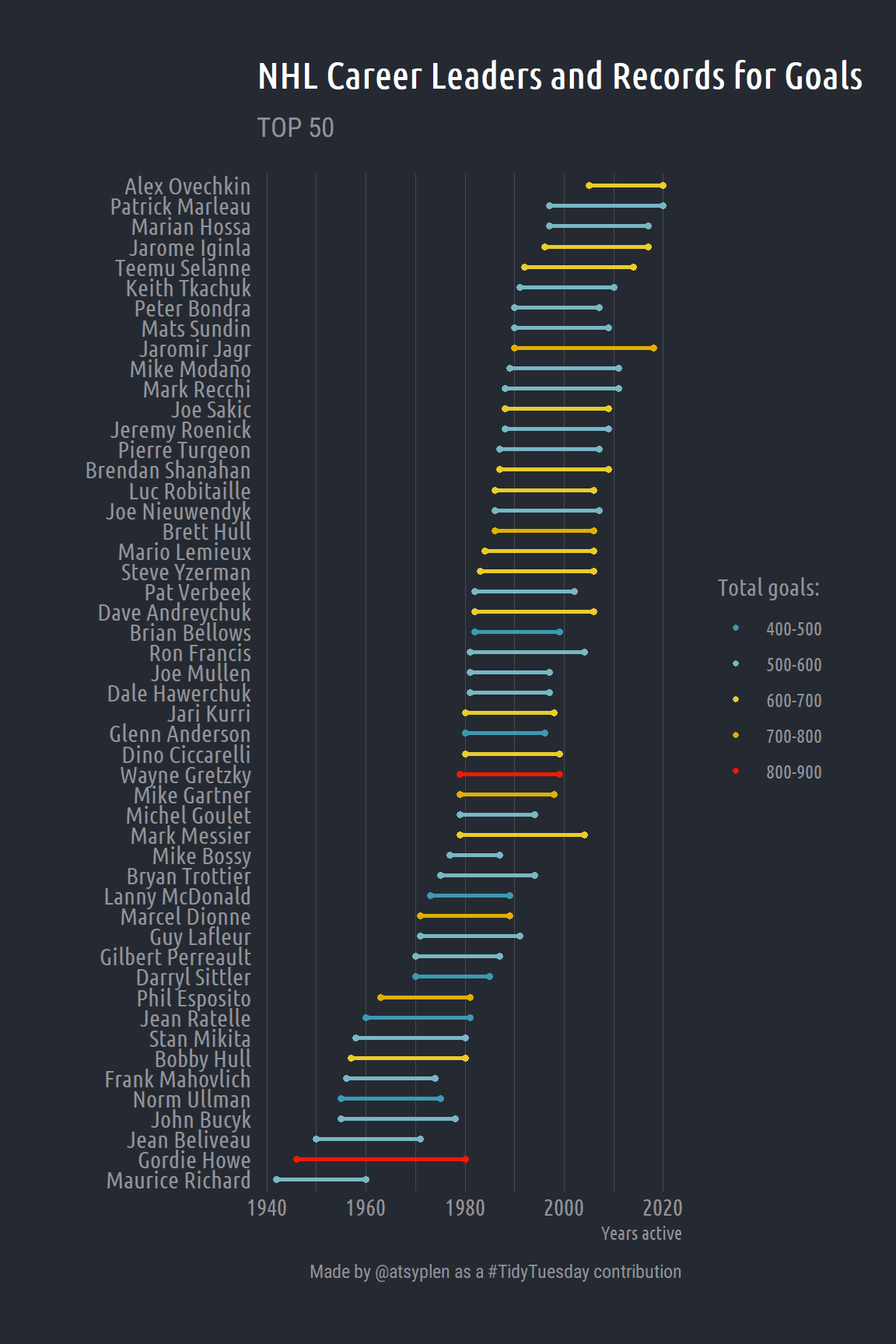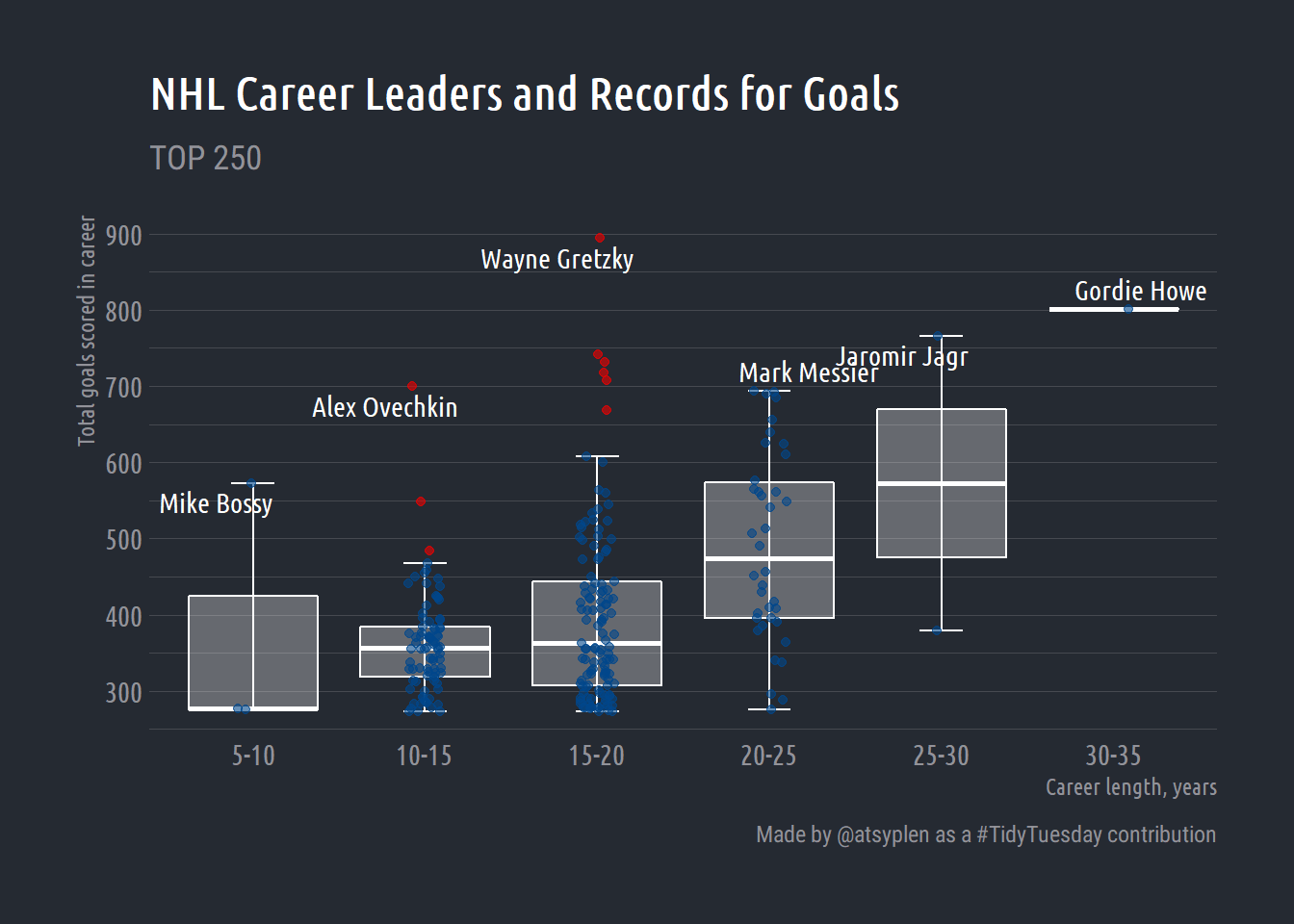# Tidy Tuesday NHL

Okay, here it is. My first contribution to the my website. I’ll try to make a very quick and simple data exploration analysis for this weeks’ Tidy Tuesday. There are three datasets available. I’m not sure that I’ll be able to analyze all of them… Let’s start from the first `top_250`.

``````library(tidyverse)
library(atslib)
library(extrafont)
library(hrbrthemes)
theme_set(hrbrthemes::theme_ft_rc(base_family = "Ubuntu Condensed"))

top_250``````
``````## # A tibble: 251 x 9
##       <dbl> <chr>   <chr>       <dbl>      <dbl> <chr>    <chr>  <chr>     <dbl>
##  1        1 Wayne ~ 1979~         894          1 /player~ https~ Retir~     1979
##  2        2 Gordie~ 1946~         801          2 /player~ https~ Retir~     1946
##  3        3 Jaromi~ 1990~         766          3 /player~ https~ Retir~     1990
##  4        4 Brett ~ 1986~         741          4 /player~ https~ Retir~     1986
##  5        5 Marcel~ 1971~         731          5 /player~ https~ Retir~     1971
##  6        6 Phil E~ 1963~         717          6 /player~ https~ Retir~     1963
##  7        7 Mike G~ 1979~         708          7 /player~ https~ Retir~     1979
##  8        8 Alex O~ 2005~         700          8 /player~ https~ Active     2005
##  9        9 Mark M~ 1979~         694          9 /player~ https~ Retir~     1979
## 10       10 Steve ~ 1983~         692         10 /player~ https~ Retir~     1983
## # ... with 241 more rows``````

This dataset contains 250 NHL & WHA career leaders and records for goals. First, we’re going to tidy our dataset a bit. What is the most interesting here is total goals really depends on the career length?

The information about years of career contains in `years` variable. It has a structure of `YYYY-YY`. First of all, let split it to `year_start` and `year_end`.

``````tidy_250 <- top_250 %>%
mutate(year_start = str_split(years, "-", simplify = T)[,1],
year_end = str_split(years, "-", simplify = T)[,2]) %>%
mutate_at(vars(year_start), ~as.numeric(.)) %>%
mutate(century = ifelse(year_start < 2000, 19, 20),
cc = str_split(year_start,
as.character(century),
simplify = T)[,2],
cc = as.numeric(cc)) %>%
mutate(year_end = case_when(year_end > cc ~ paste0(19, year_end),
TRUE ~ paste0(20, year_end))) %>%
mutate_at(vars(year_start, year_end), ~as.numeric(.)) %>%
# We need to edit millenials ))
mutate(year_end = ifelse(year_end < year_start,
year_end + 100,
year_end)) %>%
select(player, total_goals, year_start, year_end)

tidy_250``````
``````## # A tibble: 251 x 4
##    player        total_goals year_start year_end
##    <chr>               <dbl>      <dbl>    <dbl>
##  1 Wayne Gretzky         894       1979     1999
##  2 Gordie Howe           801       1946     1980
##  3 Jaromir Jagr          766       1990     2018
##  4 Brett Hull            741       1986     2006
##  5 Marcel Dionne         731       1971     1989
##  6 Phil Esposito         717       1963     1981
##  7 Mike Gartner          708       1979     1998
##  8 Alex Ovechkin         700       2005     2020
##  9 Mark Messier          694       1979     2004
## 10 Steve Yzerman         692       1983     2006
## # ... with 241 more rows``````

Great! Now we have a very tidy dataframe. However, if we are going to plot career length of all 250 players, it can be a total mess on the plot. Let’s select only first 50 players using `dplyr::top_()`.

``````tidy_50 <- tidy_250 %>%
top_n(50, total_goals)``````

I prefer to use discrete color scale instead of continuous. So I’m going to convert `total_goals` into factor. I really like to do it interactively with a new questionr package! So easy!

``````## Cutting tidy_50\$total_goals into tidy_50\$total_goals_rec
tidy_50\$total_goals_rec <- cut(tidy_50\$total_goals,
include.lowest=TRUE,  right=TRUE,
breaks = c(400, 500, 600, 700, 800, 900),
labels = c("400-500",
"500-600",
"600-700",
"700-800",
"800-900"))``````

Okay, so everything is ready for a plot! Please, notice, that I’ve already set the `ggplot` theme in the first `r` chunk via `theme_set`. I prefer to use dark themes everywhere so for this post I’ll also use a dark one. Bob Rudis (hrbrmstr) has created a really nice one in his hrbrthemes.

``````library(ggalt)
library(paletteer)

tidy_50 %>%
mutate(len = year_end - year_start) %>%
mutate(player = fct_reorder(player, year_start)) %>%
ggplot(aes(x = year_start,
xend = year_end,
y = player,
group = player)) +
ggalt::geom_dumbbell(aes(color = total_goals_rec),
size_x = 1.3,
size_xend = 1.3,
size = 1) +
labs(x = "Years active", y = "",
title = "NHL Career Leaders and Records for Goals",
subtitle = "TOP 50",
caption = "Made by @atsyplen as a #TidyTuesday contribution") +
paletteer::scale_color_paletteer_d(name = "Total goals:",
"wesanderson::Zissou1") +
theme(panel.grid.major.y = element_blank())``````Let’s create a bit more meaningful plot. It’s very interesting how `total_goals` are spread inside career lengths groups! For this one I’d prefer a boxlplot.

``````tidy_250 %>%
mutate(len = year_end - year_start) %>%
mutate(len = cut(len,
include.lowest=TRUE,  right=TRUE,
breaks = c(5, 10, 15, 20, 25, 30, 35, 40),
labels = c(
"5-10",
"10-15",
"15-20",
"20-25",
"25-30",
"30-35",
"35-40"
))) %>%
group_by(len) %>%
mutate(outlier.high = total_goals > quantile(total_goals, .75, na.rm = T) + 1.50*IQR(total_goals, na.rm = T),
outlier.low = total_goals < quantile(total_goals, .25, na.rm = T) - 1.50*IQR(total_goals, na.rm = T),
outlier.color = case_when(outlier.high ~ "red",
outlier.low ~ "steelblue",
outlier.low == F | outlier.high == F ~ "black")) %>%
ggplot(aes(x = len, y = total_goals)) +
stat_boxplot(geom ='errorbar',
width = .25,
color = "white") +
geom_boxplot(outlier.shape = NA,
color = "white",
fill = "white",
alpha = .3) +
geom_jitter(aes(color = outlier.color),
width = .1,
alpha = .6,
show.legend = F) +
scale_y_continuous(breaks = seq(100, 900, 100),
labels = seq(100, 900, 100)) +
ggrepel::geom_text_repel(data = . %>% group_by(len) %>%
filter(total_goals == max(total_goals)),
aes(label = player), segment.colour = "white",
color = "white", family = "Ubuntu Condensed") +
ggsci::scale_color_lancet() +
labs(y = "Total goals scored in career",
x = "Career length, years",
title = "NHL Career Leaders and Records for Goals",
subtitle = "TOP 250",
caption = "Made by @atsyplen as a #TidyTuesday contribution") +
theme(panel.grid.major.x = element_blank())``````##### Anatoly Tsyplenkov
###### Junior Scientist

My research interests include sediment budget, soil erosion and mountain hydrology